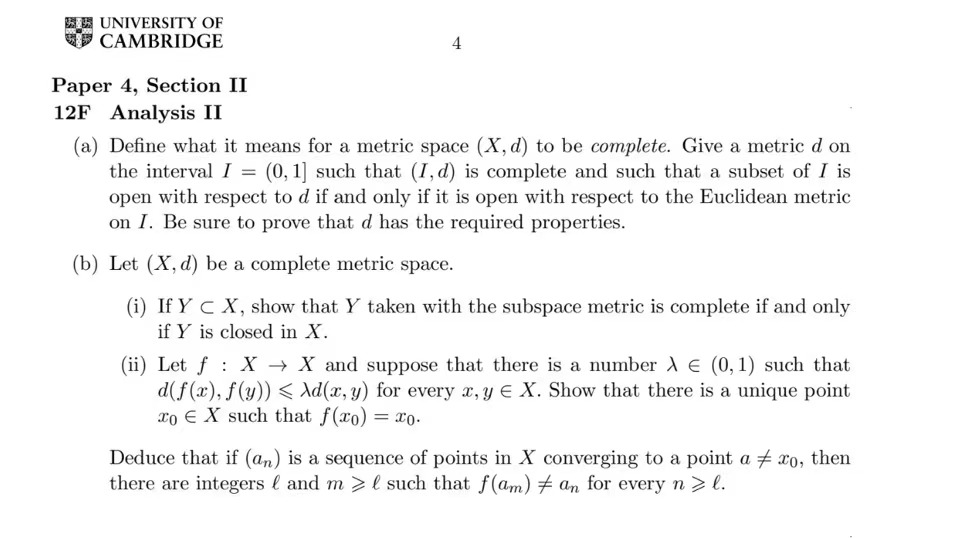### 解决全部问题

(a) Define what it means for a metric space (X. d) to be complete. Give a metric d on
the interval I = (0,1 such that (I,d) is complete and such that a subset of I is
open with respect to d if and only if it is open with respect to the Euclidean metric
on I. Be sure to prove that d has the required properties.
(b) Let (X, d) be a complete metric space.
(i) IfY C X, show that Y taken with the subspace metric is complete if and only
if Y is closed in X.
(ii) Let f: X › X and suppose that there is a number 1 € (0,1) such that
d(F(2), f(3)) < Ad(2, y) for every 2, y € X. Show that there is a unique point
20 € X such that f(20)